# SAT II Physics : Doppler Effect

## Example Questions

### Example Question #2 : Waves

A train blows its whistle as it approaches a station at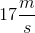. If the sound emitted by the whistle has a frequency of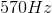, what is the frequency of the sound heard by the people at the station?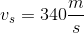Possible Answers: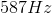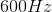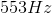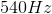Correct answer:Explanation:

Wave frequency is distorted when the observer or source is in motion based on the Doppler effect. The equation for the Doppler effect is:Terms in the numerator are summed if the observer moves toward the source. Terms in the denominator are summed if the source is moving away from the observer.

In this question, the train (source) is moving toward the observer. This means that the denominator terms will be subtracted. The velocity of the observer is zero, giving us our final equation: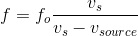Use the given values for the emitted frequency, velocity of the train, and speed of sound to solve this equation.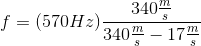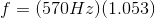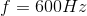### Example Question #1 : Doppler Effect

A dog is stationary in someone's front yard and barks at a frequency of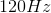. Which of the following could explain why an observer hears a frequency of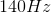?

Possible Answers:

Both the dog and the observer have the same velocity

The observer is traveling in circular path with the dog at the center of the circle

The observer is moving towards the dog

The observer is moving away from the dog

Both the dog and the observer have the same acceleration

Correct answer:

The observer is moving towards the dog

Explanation:

The doppler effect states that the observed frequency depends on the frequency produced, and the relative velocities of the observer and sound source: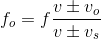To increase the observed frequency the sound source and the observer must be moving towards each other. Since the dog is stationary, therefore the observer must be moving towards the dog.

### All SAT II Physics Resources